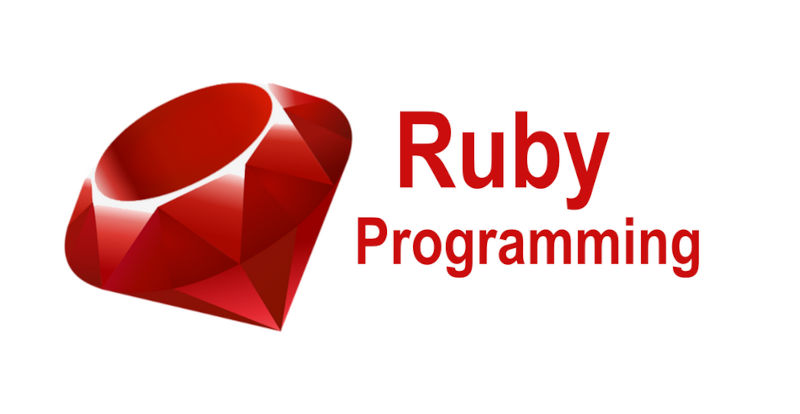#### Statistics In RubyWith users demanding more sophisticated dashboards and reports in this ocean of Data Science and Artificial Intelligence I thought it would be prudent to review how to calculate some basic statistical functionality in Ruby. First, let me point out all the code shown below should work with Ruby 2.7 and above. All the examples are in IRB. I am not here to write your code, but I do want to show you how to do the math in Ruby. Let's get started!

Calculating the Average (Mean)
Unfortunately, Ruby does not provide a native method to generate an average (mean) value from an array of integers. Its built-in Math library focuses on more complex calculations and there’s no built-in #average or #mean method on Array.

With this knowledge, we now need to create some code to do this. To calculate the mean, we need to use the Array#sum and Array#size methods in our calculation.

```irb(main):003:0> myArray = [1,2,3,4,5,6,7,8,9,10,11,12] irb(main):004:0> myArray.sum(0.0) / myArray.size => 6.5```

Bingo! As you can see that was not very difficult. A couple of items to note. First you should always use the Array#sum method if you are using Ruby 2.4 or later. Earlier Ruby language versions can use the Array#inject which is much slower than the Array#sum method.

On a final note about calculation the mean, Rails Active Record includes an #average method. It’s used to perform this calculation on numerical columns in your database using SQL. If that is your specific use case, you should definitely use that method instead.

Calculating the Median
The median is the middle number in a set of data when the data is arranged in ascending (this is more common) or descending order. If there are an even number of values in the data set, the median is the arithmetic mean of the two middle numbers. Thus, the median separates the lower and higher half of a data set. Let's get right to it!

```irb(main):001:0> irb(main):001:0> myArray = [1,2,3,4,5,6,7,8,9,10,11,12] irb(main):002:0> mySortedArray = myArray.sort # Required: data set must be sorted irb(main):003:0> myMidpoint = myArray.length / 2 # Find the midpoint array index irb(main):004:1* if myArray.length.even? irb(main):005:1* # median is mean of two values around the midpoint if even irb(main):006:1* mySortedArray[myMidpoint -1, 2].sum / 2.0 irb(main):007:1* else irb(main):008:1* mySortedArray[myMidpoint] irb(main):009:0> end => 6.5```

Calculating the Mode
The Mode is the number(s) which appear most often in a set of numbers. We will be using Array#tally (added in Ruby 2.7) to find the mode of the array.

```irb(main):001:0> myArray = [1,2,3,4,4,5,6,7,8,9,10,11,12] irb(main):002:0> myTallied = myArray.tally irb(main):003:0> myTopPair = myTallied.sort_by { |_,v| v }.last(2) irb(main):004:1* if myTopPair.size == 1 irb(main):005:1* myTopPair irb(main):006:1* elseif myTopPair == myTopPair irb(main):007:1* else irb(main):008:1* myTopPair irb(main):009:0> end => 4```
In versions earlier than 2.7 the inject method was used which is substantially slower.

What is Next
If you are going to be doing a lot of these calculations you might consider using the enumerable-statistics gem which natively implements several statistical summary methods in C which substantially faster.

Go Back To Blog.